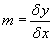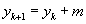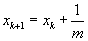←Previous Entry Next Entry→ August 17, 2005   At the risk of nitty gritty detailiosis, can we discuss the line drawing algorithms, such as the Bresenham Line-Drawing Algorithm?  Geometrically, think of a line segment as defined by its endpoints.  To plot the line on a grid, or "raster monitor," we need approximate the line by a sort "staircase."   Recall that slope isSample the line at discrete positions and determine the nearest pixel to the line for each sample.   The digital differential analyzer (DDA) scheme is to sample at unit intervals in one coordinate (say, x)  and then compute the nearest integer toHere, to be sure the pixels are connected, we assume that |m| < 1.0, but if not, we simply reverse the variables and computeThis also assumes we're plotting points from left to right so that δx = 1.  Plotting the other direction is simply a matter of  setting δx = –1 so that δy = –m.  Or, if  |m| > 1.0, take δy = –1 and δx = –1/m.    Here's how you might code this procedure (adapted from Computer Graphics with OpenGL by Hearn/Baker): void draw_lineDDA(point firstPt, point lastPt) {   int dx = lastPt.x - firstPt.x;   int dy = lastPt.y - firstPt.y;   int steps, k;   float xIncrement, yIncrement, x = firstPt.x, y = firstPt.y;   if(abs (dx) > abs (dy)) steps = abs (dx);   else steps = abs (dy);   xIncrement = float (dx) / float (steps);   yIncrement = float (dy) / float (steps);   canvas[LOC(int(x),int(y))] = black;   for(k = 0; k < steps; ++k) {     x += xIncrement;     y += yIncrement;     canvas[LOC(int(x),int(y))] = black;   } } Alternatively, Bresenham's algorithm determines which pixel should next be colored by testing the sign of an integer parameter whose value is proportional to the difference between the vertical separations of the two pixel positions from the actual line path. Starting from the left endpoint (x0, y0) of a segment, step into the next pixel column (or row, depending on whether the line is steeper than 45 degrees or not) and plot the pixel whose scan-line y value (or x value, if going the other way) is closest to the line path.   Here's the derivation.  It's pretty nifty!   Assuming that (xk, yk) is the last point in the display, how do we choose (xk+1, yk+1)?  Since the slope is less than 1, we can limit choices to (1+xk, yk)  and (1+xk, 1+yk) .  The exact y-coordinate is yk+1= m(xk+1) + b  Compare the absolute value differences dlower =  y – yk =  m(xk+1) + b – yk. dupper = (yk+1) – y  =  yk+1 – m(xk+1) – b An efficient test to determine which of these is smaller is to compute the difference of differences: dlower  – dupper =  y – yk =  2m(xk+1) + 2b – 2yk – 1 The key to the Bresenham algorithm is to realize that if we substitute m = δy/δx and multiply through by δx, then we have the relatively easily computed quantity pk as an integer decision parameter: pk = δx(dlower  – dupper)  =  2.δy.xk – 2.δx.yk + c in which c = 2.δy.+ δx(2b–1) is independent of the current pixel position, (xk, yk) , and, as such, will be eliminated in the recursive calls for pk.  Now if pk is negative then dlower  < dupper and the pixel yk is closer than 1+yk.    To get the recursive formula for pk+1 is fairly straightforward: pk+1 = 2.δy.xk+1 – 2.δx.yk+1 + c so that pk+1 – pk = 2.δy.xk+1 – 2.δx.yk+1 + c – ( 2.δy.xk – 2.δx.yk + c) = 2.δy – 2.δx.(yk+1 – yk) whence pk+1 =  pk + 2.δy – 2.δx.(yk+1 – yk)   Well, Carlsbad's calling, so I'll just slap up the code so far and be gone.  The next thing is to get this algorithm implemented and maybe compare:   /* OpenGL Template * * Look for ??? to see where your code goes! * * (Cribbed and modified from one used at Utah, * written by Kristi Potter) */ #include #include #include #include /*------------------------------------------ * Standard functions and callbacks *------------------------------------------ */ /* Initialize GL (defined below) */ void initGL(); /* Called while program is idle */ void idle(); /* Put your draw functions here */ void redraw(); /* All functions for display, calls redraw */ void display(); /* Called when a key is pressed */ void keyEvent(unsigned char key,int x,int y); /* Called when mouse button pressed */ void mouse(int button,int state,int x,int y); /* Called when the mouse moves */ void mouseMotion(int x,int y); /*---------------------------------------------------------- * USER DATA TYPES *---------------------------------------------------------- */ /* The location of a single point */ typedef struct { int x, y; } point; /* Struct to hold a single pixel (as three color bytes) */ typedef struct { unsigned char r, g, b; /* use 256 colors */ } rgb_pixel; /*-------------------------------------- * USER FUNCTION FORWARD DECLARATIONS *-------------------------------------- */ void draw_lineDDA(point p0, point p1); void fill_triangle(point a, point b, point c); /*----------------------------------------------------------- * GLOBAL VARIABLES *----------------------------------------------------------- */ /* Dimensions of the screen */ #define SCREENWIDTH 600 #define SCREENHEIGHT 500 const int screenWidth = SCREENWIDTH; const int screenHeight = SCREENHEIGHT; /*------------------------------------- * USER GLOBAL VARIABLES */ const rgb_pixel bgcolor = {210, 210, 255}; /* Light blue */ /* ??? other user global variables */ rgb_pixel black = {0, 0, 0}; rgb_pixel red = {255, 0, 0}; rgb_pixel green = {0, 255, 0}; /*------------------------------------------------- * Pixel buffer representing the whole picture * The LOC macro converts (x,y) to an index into this buffer */ rgb_pixel canvas[SCREENWIDTH * SCREENHEIGHT]; #define LOC(X,Y) ((Y)*screenWidth + (X)) /*--------------------------------------------------- * MAIN PROGRAM * draws model, calls phantom code */ int main(int argc, char **argv) { int i; /* Initialize glut */ glutInit(&argc,argv); /* Initialize GL */ initGL(); /* Makes each pixel the background color. */ for(i = 0; i < screenWidth*screenHeight; i++) { canvas[i] = bgcolor; } // event loop starts here glutMainLoop(); return 0; } /*---------------------------------------------------------------------- * init */ void initGL() { // use red/green/blue, double buffered glutInitDisplayMode(GLUT_DOUBLE | GLUT_RGB ); // Set Main Graphics Window Size glutInitWindowSize(screenWidth, screenHeight); // Set the main Graphics Window Position. glutInitWindowPosition(200,100); // the window name is an unsigned int that works like a handle glutCreateWindow("CS 365 Lab 1"); // initializes callbacks for glut glutDisplayFunc(display); glutMotionFunc(mouseMotion); glutIdleFunc(idle); glutMouseFunc(mouse); glutKeyboardFunc(keyEvent); } //+_+_+_+_+_+_+_+_+_+_+_+_+_+_+_+_+_+_+_+_+_+_+_+_+_+_+_+_+_+_+_+_+_+_+ // Idle // Sets up the idle function for glui stuff. //+_+_+_+_+_+_+_+_+_+_+_+_+_+_+_+_+_+_+_+_+_+_+_+_+_+_+_+_+_+_+_+_+_+_+ void idle() { // Redraw the contents of the display window glutPostRedisplay(); } //+_+_+_+_+_+_+_+_+_+_+_+_+_+_+_+_+_+_+_+_+_+_+_+_+_+_+_+_+_+_+_+_+_+_+ // redraw // Called to refresh and draw onto the screen. //+_+_+_+_+_+_+_+_+_+_+_+_+_+_+_+_+_+_+_+_+_+_+_+_+_+_+_+_+_+_+_+_+_+_+ void redraw() { //glPushMatrix(); /* * Put all drawing stuff here. * For this assignment we just dump our pixel map to the window */ /* Rows of pixel data are all packed together, no word-alignment */ glPixelStorei(GL_UNPACK_ALIGNMENT, 1); /* Draws the canvas array to the image buffer. */ glDrawPixels((GLsizei)screenWidth, (GLsizei)screenHeight, GL_RGB, GL_UNSIGNED_BYTE, canvas); // glPopMatrix(); } //+_+_+_+_+_+_+_+_+_+_+_+_+_+_+_+_+_+_+_+_+_+_+_+_+_+_+_+_+_+_+_+_+_+_+ // display // Sets and refreshes the window and swaps buffers. //+_+_+_+_+_+_+_+_+_+_+_+_+_+_+_+_+_+_+_+_+_+_+_+_+_+_+_+_+_+_+_+_+_+_+ void display() { // Clear the buffers glClear(GL_COLOR_BUFFER_BIT ); //Load the projection matrix glMatrixMode( GL_PROJECTION ); // Set the projection matrix to the identity matrix glLoadIdentity(); /* Simplest Orthographic 2D projection */ gluOrtho2D(-1.0, 1.0, -1.0, 1.0); redraw(); // Swap the double buffers glutSwapBuffers(); // Flush all drawing to the screen glFlush(); } //+_+_+_+_+_+_+_+_+_+_+_+_+_+_+_+_+_+_+_+_+_+_+_+_+_+_+_+_+_+_+_+_+_+_+ // keyEvent // Registers specific input characters from the keyboard. //+_+_+_+_+_+_+_+_+_+_+_+_+_+_+_+_+_+_+_+_+_+_+_+_+_+_+_+_+_+_+_+_+_+_+ void keyEvent(unsigned char key,int x,int y) { switch (key) { // Quit case 'Q': case 'q': exit(0); break; default: //do nothing on the default break; } /* ??? Add other keyboard events to the above switch as needed */ glutPostRedisplay(); } //+_+_+_+_+_+_+_+_+_+_+_+_+_+_+_+_+_+_+_+_+_+_+_+_+_+_+_+_+_+_+_+_+_+_+ // mouse // Registers when the mouse buttons are pressed. //+_+_+_+_+_+_+_+_+_+_+_+_+_+_+_+_+_+_+_+_+_+_+_+_+_+_+_+_+_+_+_+_+_+_+ int i = 1; point triangle; void mouse(int button,int state,int x,int y) { if(button == GLUT_LEFT_BUTTON && state == GLUT_DOWN) { /* ??? Here is where you accumulate triangle points * and draw a triangle when you get all three points. */ //glColor3f(1.0,1.0,0.0); if(i%3 == 1) { triangle.x = x; triangle.y = screenHeight - y; canvas[LOC(x,screenHeight - y)] = red; } if(i%3 == 2) { triangle.x = x; triangle.y = screenHeight - y; draw_lineDDA(triangle,triangle); /*glBegin(GL_LINES); glVertex2iv(triangle); glVertex2iv(triangle); glEnd();*/ } if(i%3 == 0) { triangle.x = x; triangle.y = screenHeight - y; draw_lineDDA(triangle,triangle); draw_lineDDA(triangle,triangle); //printf("color = %s\n", "black"); //canvas[LOC(x,screenHeight - y)] = black; /*glBegin(GL_TRIANGLES); glVertex2iv(triangle); glVertex2iv(triangle); glVertex2iv(triangle); glEnd();*/ } ++i; printf("i = %d\n", i); } if(button == GLUT_LEFT_BUTTON && state == GLUT_UP) { } if(button == GLUT_RIGHT_BUTTON && state == GLUT_DOWN) { /* ??? Make a note that this button is down */ } if(button == GLUT_RIGHT_BUTTON && state == GLUT_UP) { /* ??? Make a note that this button is back up */ } } //+_+_+_+_+_+_+_+_+_+_+_+_+_+_+_+_+_+_+_+_+_+_+_+_+_+_+_+_+_+_+_+_+_+_+ // mouseMotion // Registers when the mouse is moved. //+_+_+_+_+_+_+_+_+_+_+_+_+_+_+_+_+_+_+_+_+_+_+_+_+_+_+_+_+_+_+_+_+_+_+ void mouseMotion(int x,int y) { if ((x < 0) | (x >= screenWidth) | (y < 0) | (y >= screenHeight)) return; /* ??? Here insert code to move triangle if (x,y) is within * a triangle and the proper mouse button is down */ } /* Define these functions??? void draw_line(point p0, point p1); void fill_triangle(point a, point b, point c); */ void draw_lineDDA(point firstPt, point lastPt) { int dx = lastPt.x - firstPt.x; int dy = lastPt.y - firstPt.y; int steps, k; float xIncrement, yIncrement, x = firstPt.x, y = firstPt.y; if(abs (dx) > abs (dy)) steps = abs (dx); else steps = abs (dy); xIncrement = float (dx) / float (steps); yIncrement = float (dy) / float (steps); canvas[LOC(int(x),int(y))] = black; for(k = 0; k < steps; ++k) { x += xIncrement; y += yIncrement; canvas[LOC(int(x),int(y))] = black; } } void draw_lineBres(point firstPt, point lastPt) { ...to be finished...   Aedh Wishes for the Clothes of Heaven - WBYeats Had I the heavens' embroidered cloths, Enwrought with golden and silver light, The blue and the dim and the dark cloths Of night and light and the half light, I would spread the cloths under your feet: But I, being poor, have only my dreams; I have spread my dreams under your feet; Tread softly because you tread on my dreams.